# Fundamentals of Physics Study Set 2

Physics & Astronomy

## Quiz 16 : Waves IStudy FlashcardsLooking for Introductory Physics Homework Help?

## Quiz 16 :Waves I

Question TypeThree traveling sinusoidal waves are on identical strings, with the same tension.The mathematical forms of the waves are y1(x,t)= ymsin(3x - 6t), y2(x,t)= ymsin(4x - 8t), and y3(x,t)= ymsin(6x - 12t), where x is in meters and t is in seconds.Match each mathematical form to the appropriate graph below.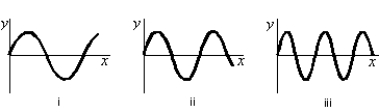Free
Multiple Choice

A

Tags
Choose question tagFor a transverse wave on a string the string displacement is described by y(x,t)= f(x-at)where f is a given function and a is a positive constant.Which of the following does NOT necessarily follow from this statement?
Free
Multiple Choice

E

Tags
Choose question tagA wave is described by y(x,t)= 0.1 sin(3x + 10t), where x is in meters, y is in centimeters and t is in seconds.The angular wave number is:
Free
Multiple Choice

E

Tags
Choose question tagThis plot shows the displacement of a string as a function of time, as a sinusoidal wave travels along it.Which letter corresponds to the amplitude of the wave?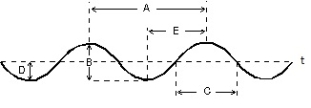Multiple Choice
Tags
Choose question tagIn the diagram below, the interval PQ represents: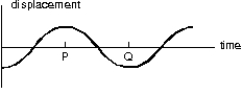Multiple Choice
Tags
Choose question tagA traveling sinusoidal wave is shown below.At which point is the motion 180 out of phase with the motion at point P?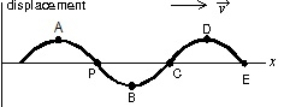Multiple Choice
Tags
Choose question tagThis plot shows the displacement of a string as a function of time, as a sinusoidal wave travels along it.Which letter corresponds to the period of the wave?Multiple Choice
Tags
Choose question tagThe displacement of a string is given by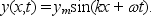The wavelength of the wave is:
Multiple Choice
Tags
Choose question tagA wave is described by y(x,t)= 0.1 sin(3x - 10t), where x is in meters, y is in centimeters and t is in seconds.How long does it take the wave to travel 2.0 m?
Multiple Choice
Tags
Choose question tagThe displacement of a string is given by y(x,t)= ymsin(kx + t). The speed of the wave is:
Multiple Choice
Tags
Choose question tagWhat is the difference between transverse and longitudinal waves?
Multiple Choice
Tags
Choose question tagThe displacement of a string carrying a traveling sinusoidal wave is given by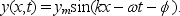At time t = 0 the point at x = 0 has velocity v0 and displacement y0.The phase constant is given by tan=:
Multiple Choice
Tags
Choose question tagTwo waves are traveling on two different strings.The displacement of one is given by y1(x,t)= ymsin(kx + t)and of the other by y2(x,t)= ymsin(kx + t + φ).What is the difference between these two waves?
Multiple Choice
Tags
Choose question tagA wave is described by y(x,t)= 0.1 sin(3x - 10t), where x is in meters, y is in centimeters and t is in seconds.The angular frequency is:
Multiple Choice
Tags
Choose question tagThe displacement of a string carrying a traveling sinusoidal wave is given by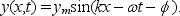At time t = 0 the point at x = 0 has a velocity of 0 and a positive displacement.The phase constant is:
Multiple Choice
Tags
Choose question tagThe displacement of a string carrying a traveling sinusoidal wave is given by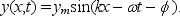At time t = 0 the point at x = 0 has a displacement of 0 and is moving in the positive y direction.The phase constant is:
Multiple Choice
Tags
Choose question tagWhat are the three main types of waves?
Multiple Choice
Tags
Choose question tagA sinusoidal wave is traveling toward the right as shown.Which letter correctly labels the wavelength of the wave?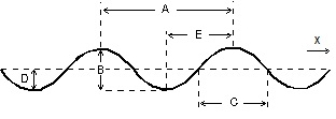Multiple Choice
Tags
Choose question tagA sinusoidal wave is traveling toward the right as shown.Which letter correctly labels the amplitude of the wave?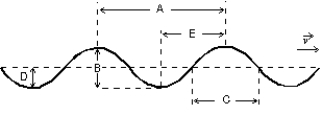Multiple Choice